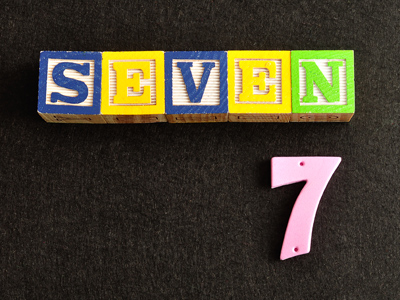7 is a prime number. It can only be divided exactly by itself and by 1.

# Properties of Numbers 2 (Difficult)

This is the second quiz in the Difficult section of our Eleven Plus maths series on Properties of Numbers. In previous quizzes we learned about prime numbers and multiples. In this one, we continue to look at those, but also some others such as factors.

Just to remind you before we start:

• Square numbers are the result of multiplying a number by itself
• Prime numbers can only be divided exactly by 1 and themselves
• Multiples are numbers that can be divided exactly by another without leaving a remainder
• Factors are numbers that divide exactly into another without leaving a remainder

Now you’ve refreshed your memory, it’s time for the quiz. Good luck!

1.
What is the smallest square number?
-2
0
1
2
0 is not considered a square number because a square that measures 0 cm x 0 cm is not a square – in fact, it couldn’t exist!
Negative numbers cannot be squares because if you multiply two negatives the result is a positive
2.
What is a factor?
A number which is the result of multiplying a number by itself
A number that can only be divided exactly by itself or by 1
A number that may be divided exactly by another number
A number that divides exactly into another number
The factors of a number are the numbers that divide exactly into it, for example:
The number 24 has 8 factors: 1, 2, 3, 4, 6, 8, 12, 24
24 x 1 = 24, 12 x 2 = 24, 3 x 8 = 24 and 4 x 6 = 24
3.
A square number is the result of multiplying a number by itself. The square of an even number will always be…?
A prime number
An odd number
An even number
Either an odd or an even number
2 x 2 = 4 (an even number), 4 x 4 = 16 (an even number), 6 x 6 = 36 (an even number)… if you want to test the idea further, then try working out the squares of some other even numbers
4.
Which number is the odd man out: 19, 27, 31, 43?
19
27
31
43
They are all odd numbers, but all of them except 27 are prime numbers too
5.
Which number is the odd man out: 16, 36, 64, 110?
16
36
64
110
They are all even numbers, but all of them except 110 are square numbers too
6.
There is only one prime number that ends in a 5. Can you name it?
5
15
25
85
Every number ending in 5 is divisible by 5. 5 itself is a prime number because it is only divisible by 1 and by 5
7.
How many square numbers are there between 1 and 100?
6
8
10
12
Here they are:
12 = 1
22 = 4
32 = 9
42 = 16
52 = 25
62 = 36
72 = 49
82 = 64
92 = 81
102 = 100
8.
Which of the following statements is wrong?
13 is a prime number
49 is a square number
The square root of 25 is 5
Square numbers can also be prime numbers
Square numbers (except 1) have at least 3 factors – their square root, 1 and themselves. Prime numbers have only 2 factors – 1 and themselves.
1 is a square number but it is not a prime number
9.
A square number is the result of multiplying a number by itself. The square of an odd number will always be…?
A prime number
An odd number
An even number
Either an odd or an even number
3 x 3 = 9 (an odd number), 5 x 5 = 25 (an odd number), 7 x 7 = 49 (an odd number)… if you want to test the idea further, then try working out the squares of some other odd numbers
10.
Which of the following statements is false?
All prime numbers are odd
Numbers which are not prime are called composite numbers
0 and 1 are not prime numbers
7 is a prime number
There is only one prime number that is even – the number 2. All other even numbers can be divided by 2.
A composite number is any number greater than 1 which is not a prime number
Author:  Frank Evans